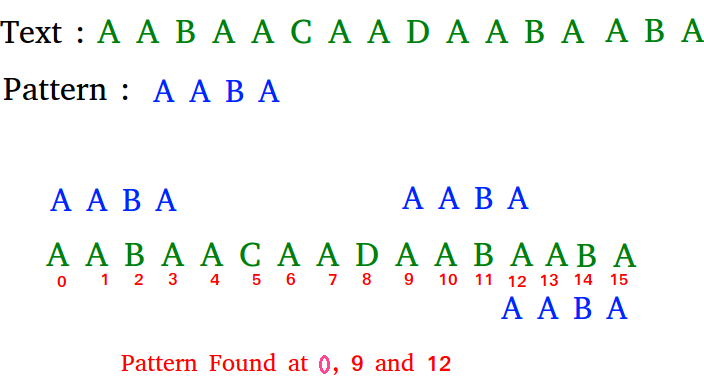# Python Program for Rabin-Karp Algorithm for Pattern Searching

• Last Updated : 30 Dec, 2020
Given a text txt[0..n-1] and a pattern pat[0..m-1], write a function search(char pat[], char txt[]) that prints all occurrences of pat[] in txt[]. You may assume that n > m.

Examples:

```Input:  txt[] = "THIS IS A TEST TEXT"
pat[] = "TEST"
Output: Pattern found at index 10

pat[] =  "AABA"
Output: Pattern found at index 0
Pattern found at index 9
Pattern found at index 12```

The Naive String Matching algorithm slides the pattern one by one. After each slide, it one by one checks characters at the current shift and if all characters match then prints the match.
Like the Naive Algorithm, Rabin-Karp algorithm also slides the pattern one by one. But unlike the Naive algorithm, Rabin Karp algorithm matches the hash value of the pattern with the hash value of current substring of text, and if the hash values match then only it starts matching individual characters. So Rabin Karp algorithm needs to calculate hash values for following strings.

1) Pattern itself.
2) All the substrings of text of length m.

## Python

 `# Following program is the python implementation of``# Rabin Karp Algorithm given in CLRS book`` ` `# d is the number of characters in the input alphabet``d ``=` `256`` ` `# pat  -> pattern``# txt  -> text``# q    -> A prime number`` ` `def` `search(pat, txt, q):``    ``M ``=` `len``(pat)``    ``N ``=` `len``(txt)``    ``i ``=` `0``    ``j ``=` `0``    ``p ``=` `0`    `# hash value for pattern``    ``t ``=` `0`    `# hash value for txt``    ``h ``=` `1`` ` `    ``# The value of h would be "pow(d, M-1)% q"``    ``for` `i ``in` `xrange``(M``-``1``):``        ``h ``=` `(h ``*` `d)``%` `q`` ` `    ``# Calculate the hash value of pattern and first window``    ``# of text``    ``for` `i ``in` `xrange``(M):``        ``p ``=` `(d ``*` `p ``+` `ord``(pat[i]))``%` `q``        ``t ``=` `(d ``*` `t ``+` `ord``(txt[i]))``%` `q`` ` `    ``# Slide the pattern over text one by one``    ``for` `i ``in` `xrange``(N``-``M ``+` `1``):``        ``# Check the hash values of current window of text and``        ``# pattern if the hash values match then only check``        ``# for characters on by one``        ``if` `p ``=``=` `t:``            ``# Check for characters one by one``            ``for` `j ``in` `xrange``(M):``                ``if` `txt[i ``+` `j] !``=` `pat[j]:``                    ``break`` ` `            ``j``+``=` `1``            ``# if p == t and pat[0...M-1] = txt[i, i + 1, ...i + M-1]``            ``if` `j ``=``=` `M:``                ``print` `"Pattern found at index "` `+` `str``(i)`` ` `        ``# Calculate hash value for next window of text: Remove``        ``# leading digit, add trailing digit``        ``if` `i < N``-``M:``            ``t ``=` `(d``*``(t``-``ord``(txt[i])``*``h) ``+` `ord``(txt[i ``+` `M]))``%` `q`` ` `            ``# We might get negative values of t, converting it to``            ``# positive``            ``if` `t < ``0``:``                ``t ``=` `t ``+` `q`` ` `# Driver program to test the above function``txt ``=` `"GEEKS FOR GEEKS"``pat ``=` `"GEEK"``q ``=` `101` `# A prime number``search(pat, txt, q)`` ` `# This code is contributed by Bhavya Jain`
Output:
```Pattern found at index 0
Pattern found at index 10
```

Please refer complete article on Rabin-Karp Algorithm for Pattern Searching for more details!

My Personal Notes arrow_drop_up